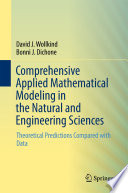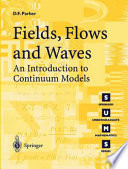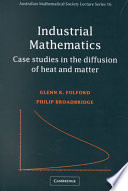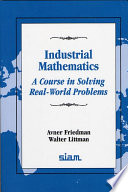### Mathematical Modelling in Engineering

Data is displayed for academic year: 2023./2024.

-

#### Study Programmes

[FER3-EN] Computing - study
Elective Courses (5. semester)
[FER3-EN] Electrical Engineering and Information Technology - study
Elective Courses (5. semester)

#### Learning Outcomes

1. Define the mathematical model.
2. Identify the influences in the process.
3. Describe a process in the language of mathematics.
4. Solve the mathematical model.
5. Analyze used methods.
6. Preispitati model.

#### Forms of Teaching

Lectures

-

Independent assignments

-

Other

-

Continuous Assessment Exam
Homeworks 0 % 30 % 0 % 0 %
Class participation 0 % 10 % 0 % 0 %
Seminar/Project 0 % 30 % 0 % 30 %
Mid Term Exam: Written 0 % 35 % 0 %
Final Exam: Written 0 % 35 %
Final Exam: Oral 10 %
Exam: Written 0 % 70 %
Exam: Oral 30 %

-

#### Week by Week Schedule

1. Introduction to mathematical modelling. Dimensional analysis.
2. Perturbation theory. Method of dominant balance.
3. Systems of ordinary differential equations, state space, equilibrium points and linearisation.
4. Regular and singular perturbations.
5. State space.
6. Herd immunity. Advanced epidemic models.
7. Predator-Prey and Lotka-Volterra model.
8. Midterm
9. Continuum modelling. Conservation Law. Heat.
10. Separation of variables. Fundamental solution and superposition.
11. Finite difference method.
12. Industrial models: steel casting.
13. Industrial models: water filtration.
14. Industrial models: laser drill.
15. Final Exam

#### LiteratureDavid J. Wollkind, Bonni J. Dichone (2018.), Comprehensive Applied Mathematical Modeling in the Natural and Engineering Sciences, SpringerDavid F. Parker (2012.), Fields, Flows and Waves, Springer Science & Business MediaGlenn Fulford, Glenn R. Fulford, Philip Broadbridge (2002.), Industrial Mathematics: Case Studies in the Diffusion of Heat and Matter, Cambridge University PressAvner Friedman, Walter Littman (1994.), Industrial Mathematics: A Course in Solving Real-World Problems, SIAM

#### General

ID 227566
Winter semester
5 ECTS
L0 English Level
L1 e-Learning
45 Lectures
0 Seminar
0 Exercises
0 Laboratory exercises
0 Project laboratory